﻿ Mass Energy Equivalence stock illustration. Illustration of symbol - 83090911

# Mass Energy Equivalence. Symbol, sign.

## Mass Energy Equivalence - Abstract Illustration

More similar stock illustrationsBack to school seamless pattern, mass energy equivalence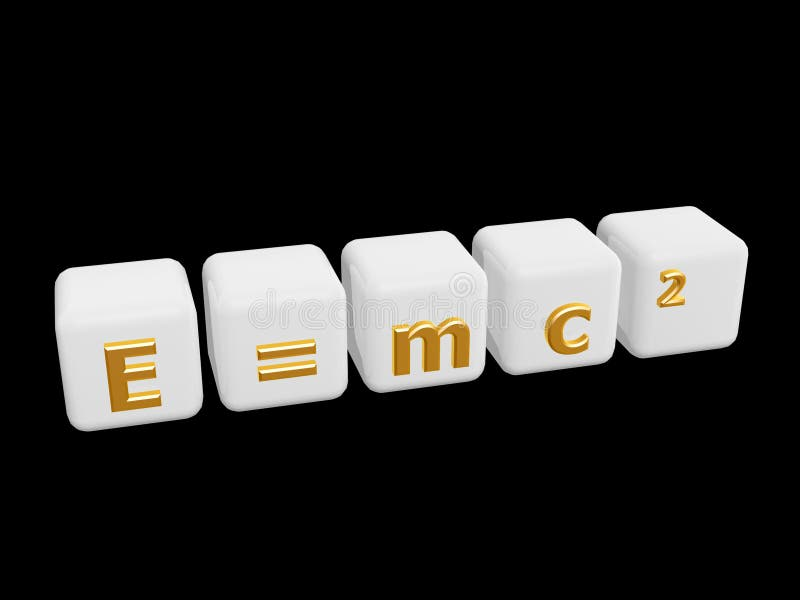Mass energy equivalence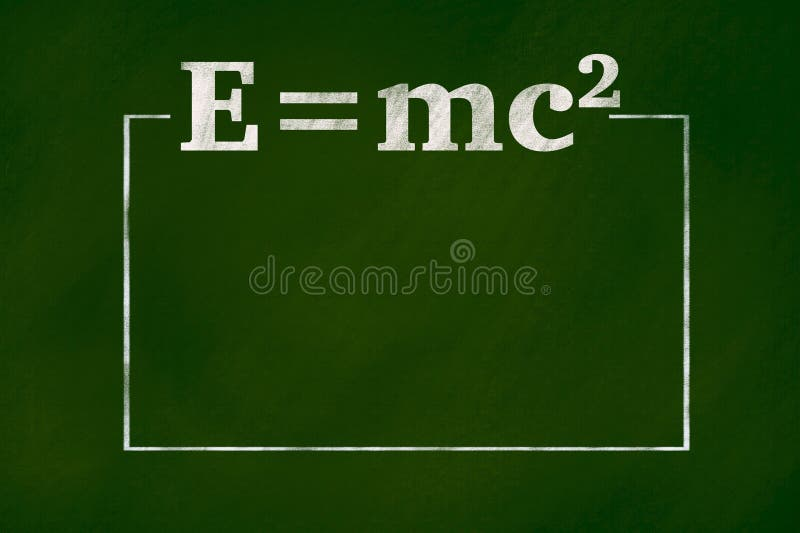mass-energy equivalent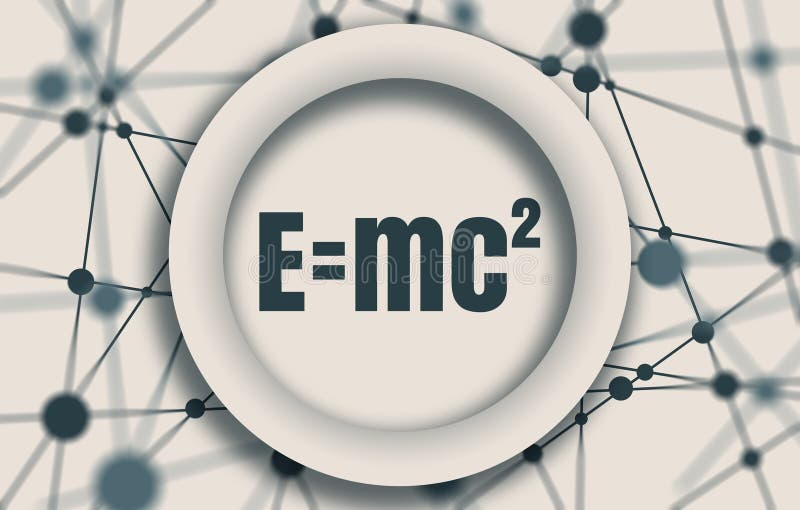Physical formula of mass and energy equivalence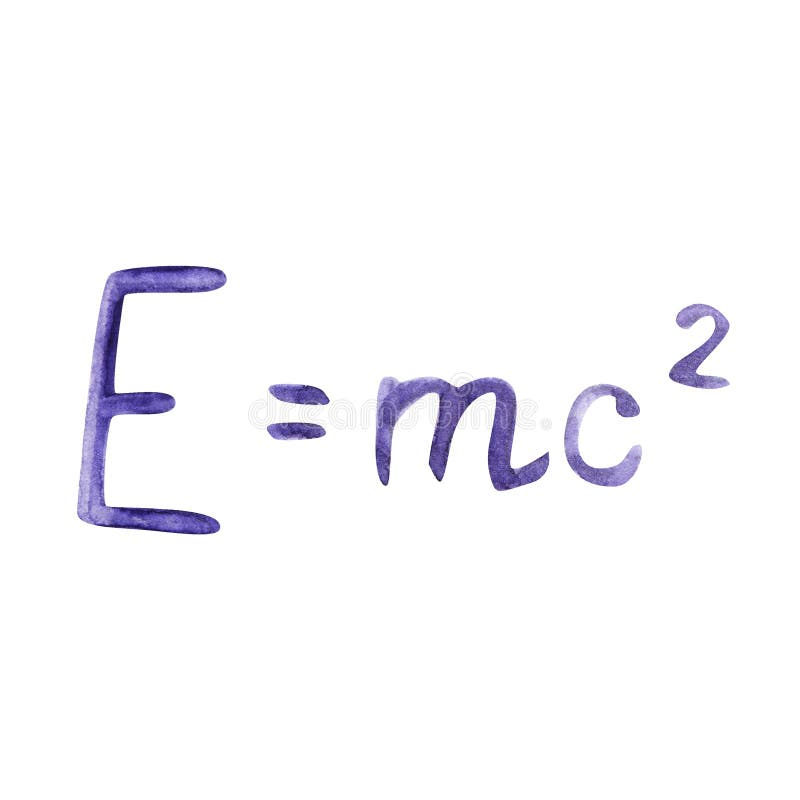Watercolor illustration drawn with formula. The famous formula E mc2. Formula expressing the equivalence of mass and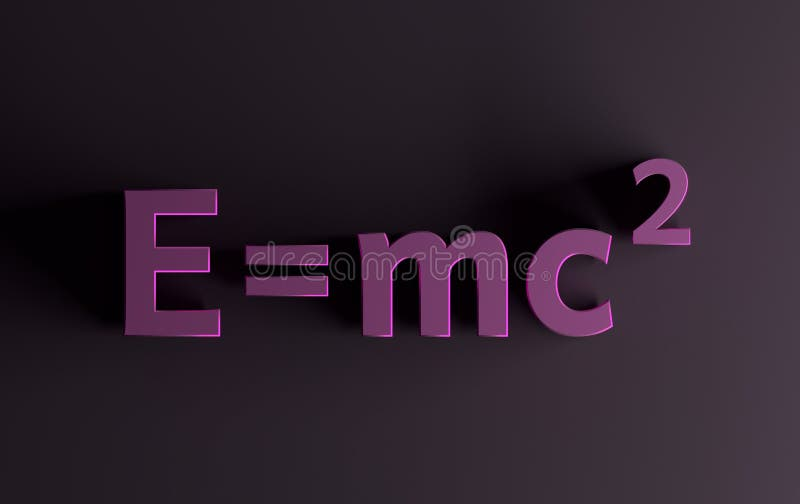Mass energy formula in pink colors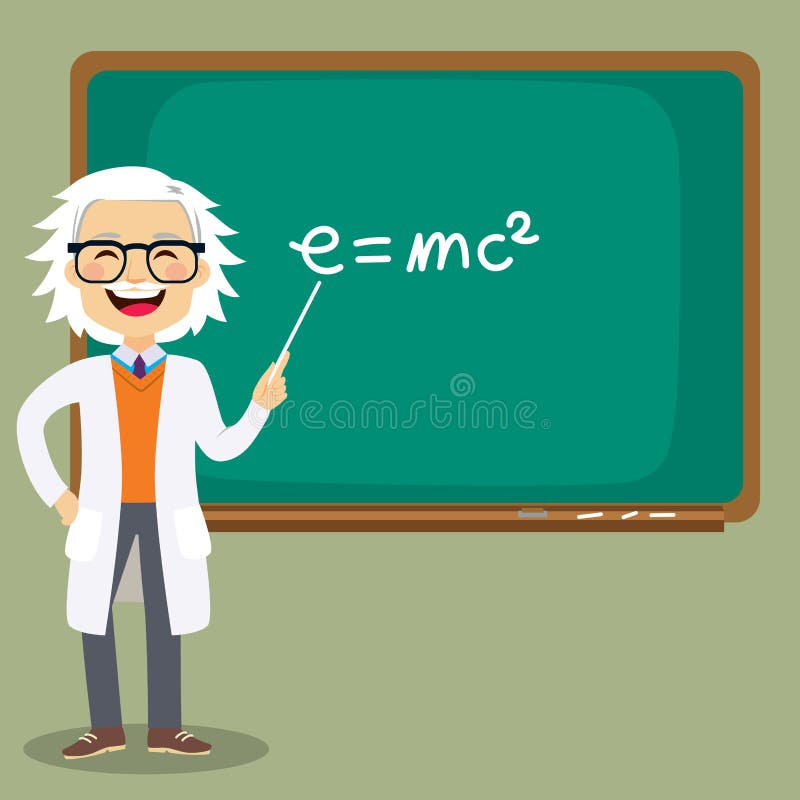Senior Science Teacher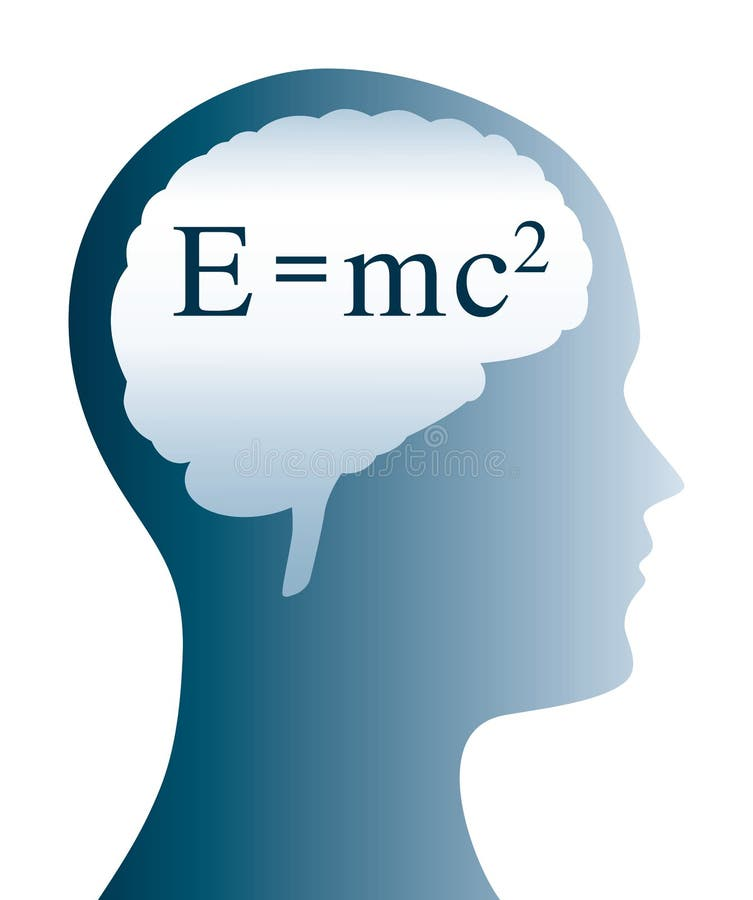E=mc2 Einstein formula in brain and head silhouette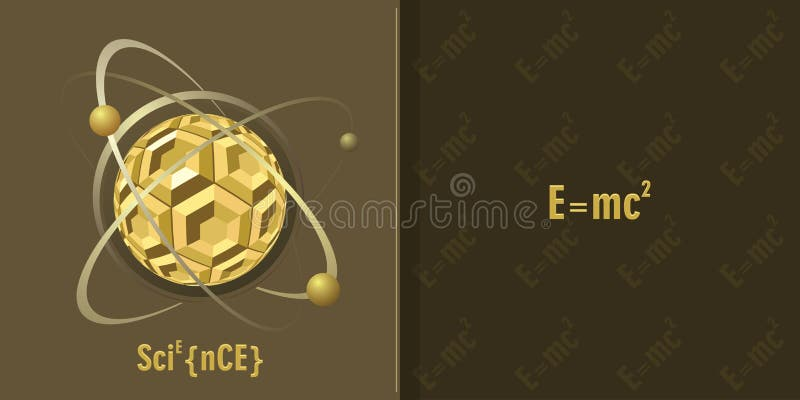Science background
More stock photos from Spgirolamo's portfolioMass Energy EquivalenceE = mc2 - Mass Energy EquivalenceMass Energy EquivalenceE = mc2 - Mass Energy EquivalenceRed Giant StarMass Energy EquivalenceMass Energy EquivalenceE = mc2 - Mass Energy EquivalenceRed Giant Star